# MALAAVIRU9.The graph above shows the polar curves r=3+ cos 0- sin(20) and r=1+sin 0. What is thearea bounded between the two polar curves?Asked 7 minutes ago

Question
4 views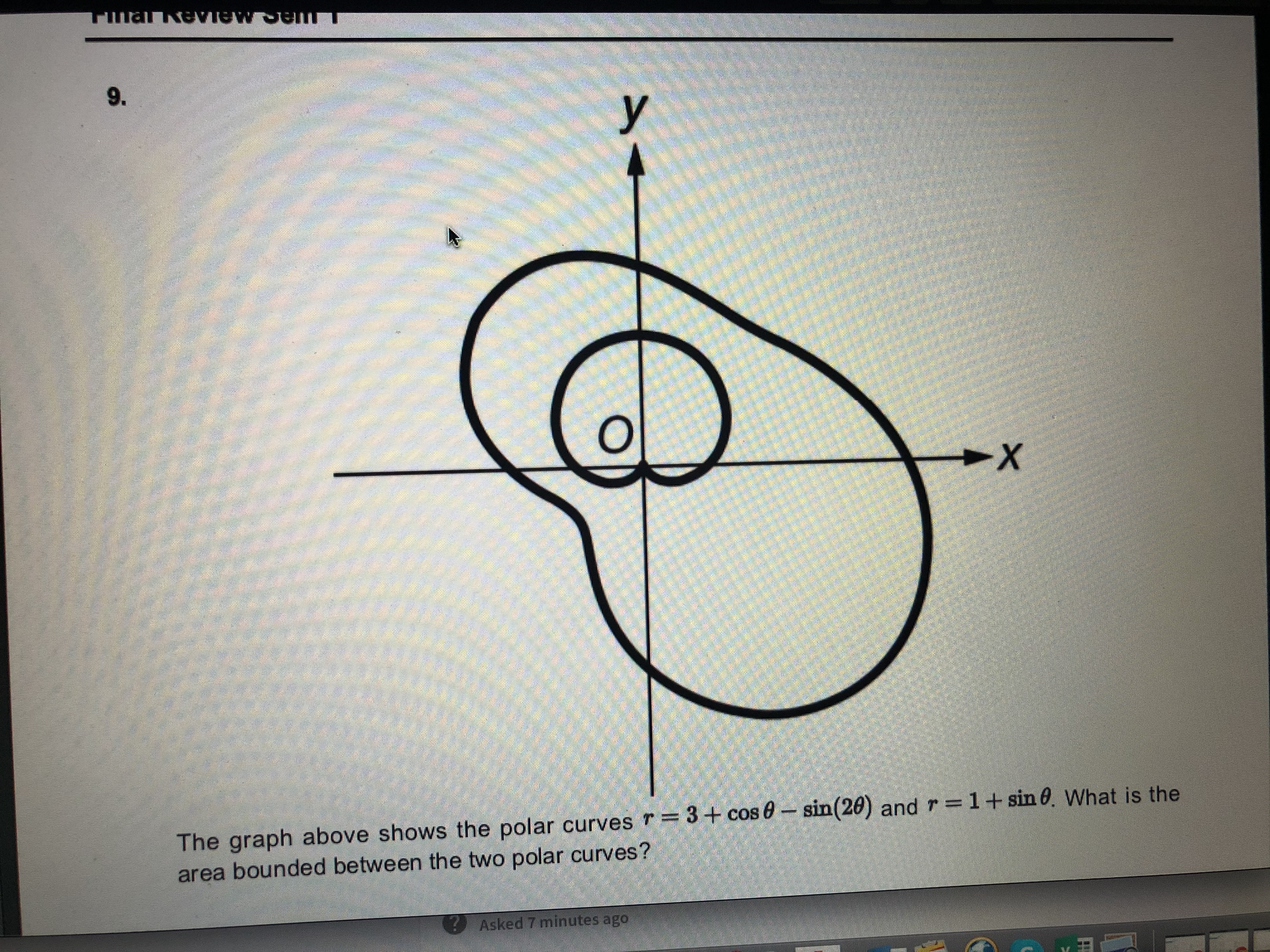help_outlineImage TranscriptioncloseMALAAVIRU 9. The graph above shows the polar curves r=3+ cos 0- sin(20) and r=1+sin 0. What is the area bounded between the two polar curves? Asked 7 minutes ago fullscreen
check_circle

Step 1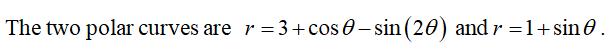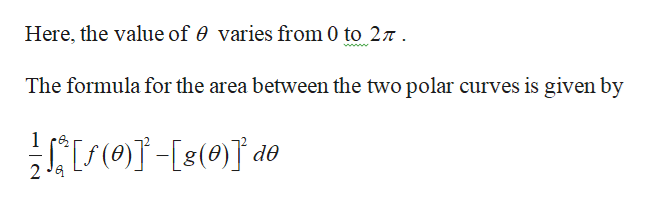help_outlineImage TranscriptioncloseHere, the value of 0 varies from 0 to 27 . The formula for the area between the two polar curves is given by (-[s()] de 2 Ja fullscreen
Step 2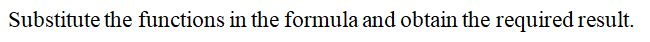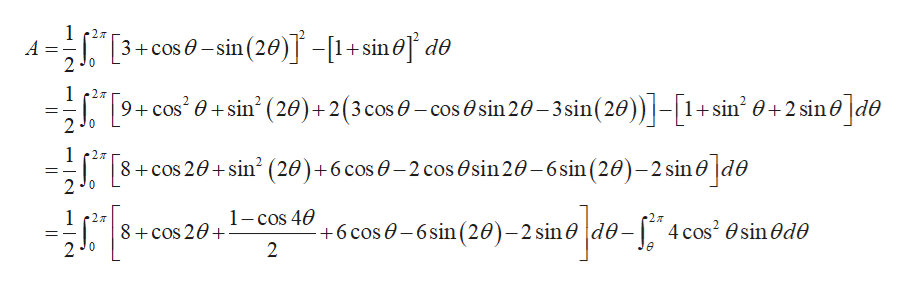help_outlineImage Transcriptionclose"[3+cos 0 – sin (20) -[1+sin@] d® 2 Jo 2л (28))]-[1+sin² 0 + 2 sin e]d® 9+ cos' 0 + sin (20)+2(3 cos 0 – cos O sin 20– 3sin(20 2 Jo в. 2л 8+cos 20+ sin? (20)+6 cos 0 – 2 cos Osin 20–6 sin (20)– 2 sin0 |d0 2 J0 но до-F 1- cos 40 2л de-| 4 cos? O sin Ode - +6 cos 0 – 6sin (20)-2 sin0 8 + cos 20+ fullscreen
Step 3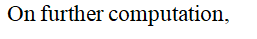...

### Want to see the full answer?

See Solution

#### Want to see this answer and more?

Solutions are written by subject experts who are available 24/7. Questions are typically answered within 1 hour.*

See Solution
*Response times may vary by subject and question.
Tagged in